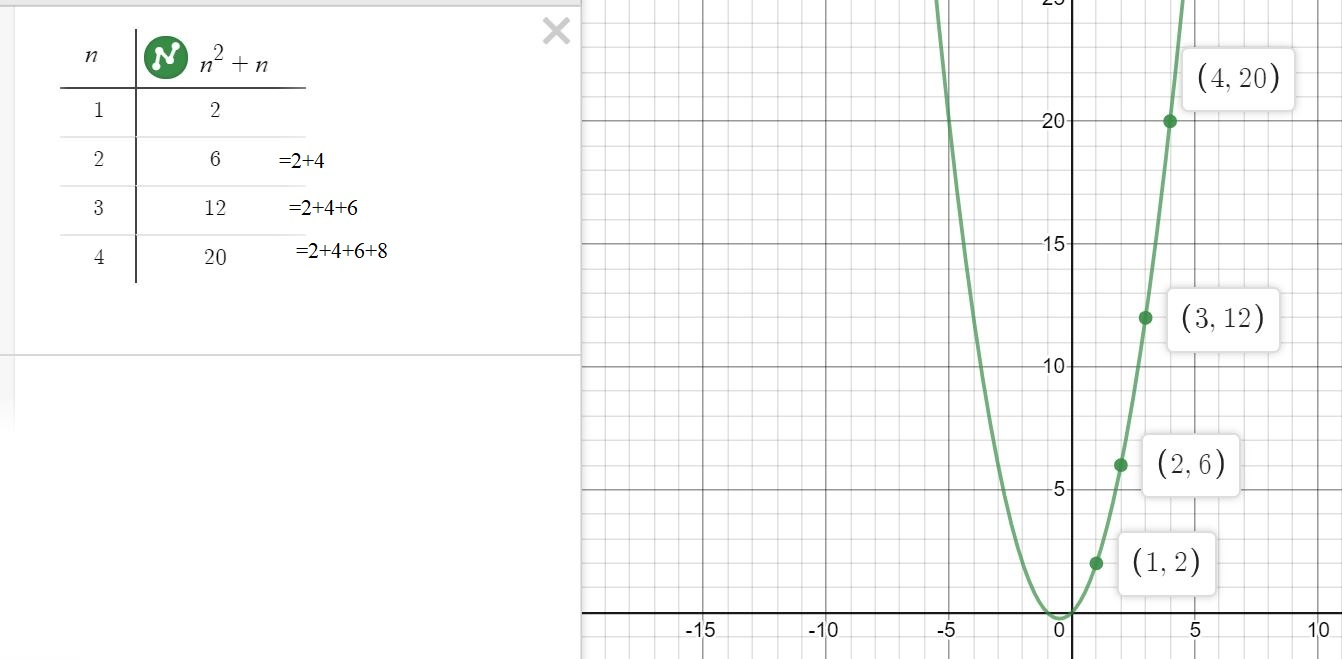# Activity – Sequences and Series

1. To understand the concept of arithmetic sequence sketch the graph of some linear functions using some graphing software ( I used DESMOS). You will observe that when the y-values will change increase/decrease with a constant number. Try to link it with the general term of an arithmetic sequence  ${u}_{n}={u}_{1}+\left(n–1\right)d$ and draw the conclusion that the coefficient of n represents the common difference and also the gradient of the line. Here is an exampleYou can sketch exponential functions of the form  to understand geometric series.

2. Sketch the graph of quadratics of the form  $y=a{n}^{2}+bn$ to understand the sum to terms of an arithmetic series. e.g.